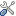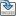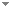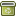# Complex Vector Formalism of Harmonic Oscillator in Geometric Algebra: Particle Mass, Spin and Dynamics in Complex Vector Space

Foundations of Physics 44 (3):266-295 (2014)

 Abstract Elementary particles are considered as local oscillators under the influence of zeropoint fields. Such oscillatory behavior of the particles leads to the deviations in their path of motion. The oscillations of the particle in general may be considered as complex rotations in complex vector space. The local particle harmonic oscillator is analyzed in the complex vector formalism considering the algebra of complex vectors. The particle spin is viewed as zeropoint angular momentum represented by a bivector. It has been shown that the particle spin plays an important role in the kinematical intrinsic or local motion of the particle. From the complex vector formalism of harmonic oscillator, for the first time, a relation between mass $m$ and bivector spin $S$ has been derived in the form $\varvec{\sigma }_3 mc^2{\mathcal {J}}_{\pm } =\lambda \Omega _{\mathbf{s}} \cdot \mathrm{{S}} {\mathcal {J}}_{\pm }$ . Where, $\Omega _{s}$ is the angular velocity bivector of complex rotations, $c$ is the velocity of light. The unit vector $\varvec{\sigma }_3$ acts as an operator on the idempotents ${\mathcal {J}}_{+}$ and ${\mathcal {J}}_{-}$ to give the eigen values $\lambda =\pm 1.$ The constant $\lambda$ represents two fold nature of the equation corresponding to particle and antiparticle states. Further the above relation shows that the mass of the particle may be interpreted as a local spatial complex rotation in the rest frame. This gives an insight into the nature of fundamental particles. When a particle is observed from an arbitrary frame of reference, it has been shown that the spatial complex rotation dictates the relativistic particle motion. The mathematical structure of complex vectors in space and spacetime is developed Keywords Zeropoint energy  Spin  Particle mass  Geometric algebra Categories (categorize this paper) ISBN(s) DOI 10.1007/s10701-014-9784-2 OptionsEdit this recordMark as duplicateExport citationFind it on ScholarRequest removal from indexRevision history

PhilArchive copy

Upload a copy of this paper     Check publisher's policy     Papers currently archived: 69,959

Setup an account with your affiliations in order to access resources via your University's proxy server
Configure custom proxy (use this if your affiliation does not provide a proxy)

## References found in this work BETA

Zitterbewegung in Quantum Mechanics.David Hestenes - 2009 - Foundations of Physics 40 (1):1-54.
On Vacuum Fluctuations and Particle Masses.M. D. Pollock - 2012 - Foundations of Physics 42 (10):1300-1328.

## Similar books and articles

On Vacuum Fluctuations and Particle Masses.M. D. Pollock - 2012 - Foundations of Physics 42 (10):1300-1328.
On Classical and Quantum Relativistic Dynamics.F. Reuse - 1979 - Foundations of Physics 9 (11-12):865-882.
Spin State Determination Using a Stern-Gerlach Device.M. I. Shirokov - 1998 - Foundations of Physics 28 (6):985-997.
Quantum Relativistic Action at a Distance.Donald C. Salisbury & Michael Pollot - 1989 - Foundations of Physics 19 (12):1441-1477.
A Classical Klein—Gordon Particle.Nathan Rosen - 1994 - Foundations of Physics 24 (11):1563-1569.
A Classical Proca Particle.N. Rosen - 1994 - Foundations of Physics 24 (12):1689-1695.